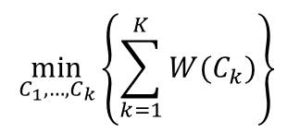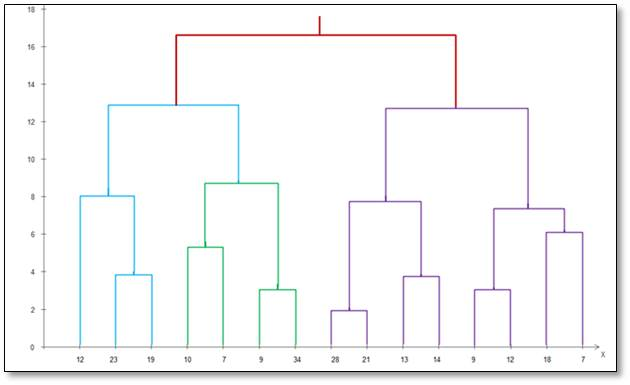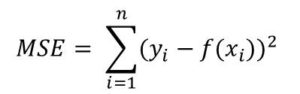# Machine Learning Questions and Answers (Questions 11 to 20)

This is Machine Learning Questions and Answer (11 to 20)

Find Question 1 to 10 here.

So let’s get started!

##### 11. Explain Clustering in your own words

First, note that clustering is an unsupervised learning technique. It is used to find sub-groups or clusters within a dataset.

When a set of observation is clustered, we partition the data into unique groups such that the data points within each of the groups share some common characteristics. At the same time, data points in different groups are different from each other.

The two main types of clustering are

##### 12. Briefly Describe k-Means Clustering

K-Means clustering seeks to partition the set of observations into K non-overlapping clusters.

The first step is to choose the number of clusters, K.

Then we define the K clusters. Let’s say C1, C2, … Ck.

These clusters should satisfy the two conditions:

All-inclusive condition: this is given by C ∪  C ∪ … ∪  Ck = {1, 2, …}. This means that all observations must belong to one of the K clusters

Non-overlapping condition: this is given by Ci ∩  Cj = Ø

The objective of k-means clustering is to minimize the within-cluster variation W(Ck) for cluster K. This is that amount  by with the data points within a particular cluster differ from each other. Therefore k-means clustering seeks to find:This formula simply means that we would like to partition the data points into K clusters so that the overall within-cluster variation, summed over all K clusters is minimum.

K-Means clustering procedure video

##### 13. What is Hierarchical Clustering?  How is it different from k-Means?

Hierarchical clustering an alternative clustering approach to K-Means. Unlike the K-Means, hierarchical clustering  does not require specifying the initial number of clusters. It produces a set of nested clusters that can be organized and visualized as a hierarchical tree. This tree is called  dendrogram.

The two types of hierarchical clustering are: Agglomerative and Divisive

Agglomerative: Begin with each data point as its own cluster. Then at each step, it merges the nearest cluster until one cluster (or k clusters) is left.

Divisive: In this case, we start with one large cluster that includes all the elements. Then at each step, we split the cluster until all each cluster contains only a single data point

##### 14. What is a Dendrogram. Give an Example

A dendrogram is a tree-like diagram obtains from the hierarchical clustering process. It  indicates the merges(or the split) carried out at each step of hierarchical clustering. The bottom of the tree are leaves which represent clusters of individual data points. As we move up the tree, the branches fuse together to for larger clusters.

An example of a dendrogram is given below:##### 15. What is Spectral Clustering?

This is a more complex clustering method that is based on the graph theory. In spectral clustering, set of nodes in a graph are identified based on  edges that connect them.

First, it uses information obtained from the spectrum(or eigenvalues) of the similarity(spatial) matrix to perform dimensionality reduction on  the data. Next, it builds the graph. Finally, it clusters the data.

(you don’t need to focus on this type, unless you want to take on more advanced studies in data science/ML)

##### 16. What clustering algorithm is better? Give reasons

The K-means clustering has a drawback of having to pre-choose the value of K. This restriction does not exist for hierarchical clustering.

Hierarchical clustering has the following limitation:

• once a decision is made to combine two clusters, the decision cannot be undone
• no clear objective function is minimized
• sometimes breaking large clusters could be complex
• tends to be more sensitive to noise than k-means

However, hierarchical clustering has the benefit of producing a set of clusters that can be visualized.

Based on these few thoughts, it then seems I would prefer the K-means clustering!

##### 17. What is bias in an Machine Learning Model

Bias is the error introduced to our model when a simple model used in situation where a more complex model would have done better.

For example, take the case of linear regression. Here a (simple) linear relationship is assumed between X and Y. But in the real world, it is likely the relationship may not really be linear. In this case, we are just being biased!!

Generally, the more the biased, the less complex the model. An less flexible the model as well.

##### 18. What is Variance a Machine Learning Model

Variance refers to the variation in the model due to the variation in the training data used to build the model. Remember that models are built using training data. Also remember that a model is simply a function or a relation that maps x to y.

So let’s assume we have  store of training set of say, 100,000 observations. Then you random take out 500 records and use to build a model f1(x). Next you take out another 1000 records and build a second model, f2(x). The difference between these two models is what is known as variance.

You can think of variance in this case as:

##### variance =  f1(x) – f2(x)

Normally, the higher the variance, the more flexible the model. The  more complex the model as well.

##### 19. What is Mean-Squared Error(MSE)

The Mean-Squared-Error is a measure of the the quality of a model. When a model makes a prediction, the MSE provide  a way to know ow well the prediction match the real data.

For example if we have a data set X = {x1, x2, …, xn}.

Each observation has a corresponding y given by Y = {y1, y2, …, yn}

So we develop a model that maps the Y values to the X values and our model is in form a function f(x). In ideal case, f(xi) should equal yi. But that is not the case in real scenario. There is always a difference between f(xi) and yi. This difference is the error.

The Mean-Square-Error defines this difference using the formula:The MSE is computed using the training dataset. Therefore it also called training MSE.

##### 20. Explain the concept of Bias/Variance Trade-off

Remember that the more the variance, the more complex the model. Similarly, the more the bias, the less complex the model. Therefore bias and variance has in inversely proportional relationship.

We don’t want a model that is too complex(much variance) such that it causes overfitting. On the other hand, we don’t want a model that has very little complexity (much bias) that it leads to underfitting. So we need to find a balance between the bias and the variance. This balance is called bias/variance trade-off. (Questions on Underfitting and overfitting are answered in Question 21 to 30)